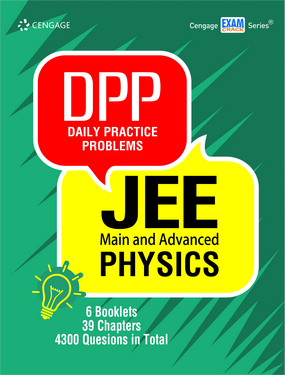###### Home
>

Test Prep### Daily Practice Problems JEE Main and Advanced: Physics

Author(s): Cengage India

ISBN: 9789353501495

Edition: 1st

#### Rs. 590

Binding: Paperback

Pages: 688

Trim Size : 279 x 216 mm

Daily Practice Problems (DPPs) are a set of 6 booklets of Physics that contain questions segregated chapterwise and topicwise. The questions have been carefully picked and set by authors understanding the need of a JEE aspirant as per their daily preparation schedule. Each chapter in a booklet is presented as a DPP containing questions of both JEE Main and Advanced level. The question types used in these DPPs are in exact lines with those that appear in JEE Main and Advanced. Detailed solutions are given to all the questions at the end of each booklet.

• A total of 4300 questions are included in 6 booklets.
• The question bank caters to both JEE Main and Advanced preparation.
• Four types of questions have been included in the DPPs – Subjective Type, Single Correct Answer Type, Multiple Correct Answers Type and Linked Comprehension Type.
• Solutions to all questions are given at the end of each booklet.

Electrostatics and Current Electricity

Chapter 1: Coulomb's Laws and Electric Field

Chapter 2: Electric Flux and Gauss’s Law

Chapter 3: Electric Potential

Chapter 4: Capacitor and Capacitance

Chapter 5: Electric Current and Circuits

Chapter 6: Electrical Measuring Instruments

Chapter 7: Heating Effects of Current

Magnetism and Electromagnetic Induction

Chapter 1: Magnetic Field and Magnetic Forces

Chapter 2: Sources of Magnetic Field

Chapter 3: Electromagnetic Induction

Chapter 4: Inductance

Chapter 5: Alternating Current

Mechanics I

Chapter 1: Unit Dimension and Measurement

Chapter 2: Basic Mathematics

Chapter 3: Vectors

Chapter 4: Motion in One Dimension

Chapter 5: Motion in Two Dimensions

Chapter 6: Newton’s Laws of Motion I

Chapter 7: Newton’s Laws of Motion II

Chapter 8: Work, Power, and Energy

Mechanics II

Chapter 1: Center of Mass

Chapter 2: Rigid Body Dynamics I

Chapter 3: Rigid Body Dynamics II

Chapter 4: Fluid Mechanics

Chapter 5: Properties of Matter

Chapter 6: Gravitation

Optics and Modern Physics

Chapter 1: Ray Optics

Chapter 2: Wave Optics

Chapter 3: Electrons, Photons, Photoelectric Effect and X-Rays

Chapter 4: Atomic Physics

Chapter 5: Nuclear Physics

Waves and Thermodynamics

Chapter 1: Thermometry, Thermal Expansion and Calorimetry

Chapter 2: Transmission of Heat

Chapter 3: Kinetic Theory of Gases

Chapter 4: Thermodynamics

Chapter 5: Linear and Angular Simple Harmonic Motion

Chapter 6: Travelling Waves

Chapter 7: Superposition and Standing Waves

Chapter 8: Sound Waves and Doppler Effect